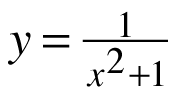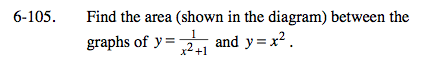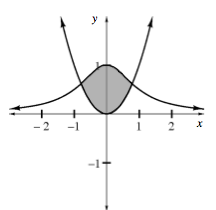### Home > CALC > Chapter 6 > Lesson 6.3.3 > Problem6-105

6-105.
1. Find the area (shown in the diagram) between the graphs ofand y = x2. Homework Help ✎Identify which function is on top and which is on the bottom.

$\text{Area between curves}=\int_{A}^{B}((\text{top function})-(\text{bottom function}))dx$

A = left intersection point
B = right intersection point